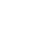# 50 Dollars An Hour – How Much A Year? (Example Budget Included)

## How Much Is 50 Dollars An Hour Per Year?

To calculate how much you will get paid per year, let’s assume you work 52 weeks of the year (with 2 weeks paid time off). If you are working a full-time job, you will be working 40 hours per week on average.

40 hours multiplied by 52 weeks is 2,080 working hours in a year.

\$50 per hour multiplied by 2,080 working hours per year is an annual income of \$104,000 per year.

## Simple Tools To Live On \$50 An Hour

[elementor-template id=”506255″]

## What If You Don’t Get Paid Time Off?

If you don’t get paid vacation, let’s assuming you are working 50 weeks of the year (with 2 weeks unpaid time off). And we’ll assume you work an average of 40 hours per week.

40 hours multiplied by 50 weeks is 2,000 working hours in a year.

Now simply multiply your hourly rate by the number of working hours in the year.

\$50 per hour multiplied by 2,000 working hours per year is an annual income of \$100,000 per year.

## How Many Working Days Are In A Year (2020)?

For a more accurate calculation, you can calculate EXACTLY how many working days are in the year.

For the year 2020, it’s a leap year, so there are 366 days in the year. Here’s how the days break down:

• 262 Weekdays
• 104 Weekend Days (woohoo!)

Assuming your are working each weekday, 8 hours per day, here is how many hours you will work:

262 work days multiplied by 8 hours per day is 2,096 working hours in 2020

\$50 an hour multiplied by 2,096 working hours is \$104,800 income per year

*This does not include any overtime hours worked

## How Much Is \$50 An Hour Per Month?

If you want to see how much \$50 an hour is a month, we need to know how many working hours there are in a month.

If we divide the total working hours in a year by 12 (months), we can see how many working hours are in a month.

2,096 hours a year divided by 12 is about 175 working hours per month on average.

So to calculate your monthly income, see below:

\$50 an hour multiplied by 175 hours per month is \$8,750 per month income on average.

## How Much Is \$50 An Hour Per Week?

If you want to break it out by week, let’s assume your working a normal 40-hour week.

So to calculate your weekly income, see below:

\$50 an hour multiplied by 40 hours per week is \$2,000 per week income.

## How Much Is \$50 An Hour Per Day?

Let’s see how much you can make per day on \$50 an hour. Assuming you are working a typical 8-hour workday, here’s the answer:

\$50 an hour multiplied by 8 hours per day is \$400 per day income.

Now that you know how much you can make per year, per month and weekly, let’s see how a typical budget can look making \$50 per hour.

## Example Budget For \$50 Per Hour

Remember, for your budget, you need to calculate the estimated take-home pay.

Taking the \$50 an hour monthly income of \$8,750, minus taxes, the estimated take home pay is \$6,390 (varies by state and paycheck deductions)

Sample Monthly Budget For \$50 An Hour:

As you can see, this budget allows for saving a decent amount (over \$600 per month), and would be great for a young family. I recommend putting the \$600+ per month into your work 401k account up to the match, and then into a Roth IRA next.

If looking at buying a house on \$50 an hour, make sure you can put 20% down and keep monthly housing costs under \$2,000 per month.

Don’t like the budget? Grab your own budget template below and plug in your numbers for your \$50 an hour budget.

1.